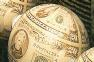Financial Calculus Book ReviewFinancial Calculus
An introduction to derivative pricing
RISK Book review

The following is an unedited review of Financial Calculus which appeared in the Journal of the Royal Statistical Society A, Vol 160, Part 2, 1997.

# Financial Calculus

## reviewed by D.C. Bowie

The stated intention of this book is to help to fill the gap between practice and theory in the rapidly expanding area of mathematical finance. Although it is a little light on the practical side on occasions (market practice is rarely mentioned and very few data are presented) it does, on the whole, achieve its aim. Certainly, in conjunction with a more practically oriented book such as that by Hull (1997), it would provide an excellent introduction to the field of derivative pricing and hedging.

The book contains six chapters (plus a `parable' which introduces the basic concept of hedging). The first chapter is an introduction to expectation pricing and arbitrage-free pricing of financial products and a brief comparison of the two. It argues convincingly and succinctly that conventional expectation-based pricing strategies are unenforceable in a complete market, in contrast with prices derived from arbitrage-free hedging strategies. In Chapter 2 the authors characterize the main issues to be dealt with in the book and outline a discrete time approach to modelling financial products and derivatives. They describe in detail a binomial branch model of stock price processes and use this to illustrate many of the mathematical concepts used later in the less intuitive realm of continuous time processes. In particular, they are insistent on the distinction between process and measure, which is clearly a key insight before tackling something like the Cameron-Martin-Girsanov (CMG) theorem which is to be found in Chapter 3. The second chapter acts, therefore, as in intuitionally easy prelude to Chapter 3 which is arguably the most valuable and important in the book.

Chapter 3 contains a careful discussion of the mathematical underpinnings required to understand the key results in financial economics. Readers who are not familiar with Brownian motion, martingales, the Radon-Nikodym derivative, the CMG change of measure theorem and Ito's lemma are taken through these results in a most accessible manner. The mathematical tools are then used to construct replicating strategies for hedging financial derivatives. The chapter culminates with a description of the Black-Scholes model and its famous pricing formula for European-style call options.

Chapter 4 extends the pricing strategies outlined in Chapter 3 to more complex and realistic financial products, including currencies, dividend paying stocks, coupon-bearing bonds and quantos. The chapter also includes a definition of tradable securities and a brief introduction to the market price of risk and risk neutral measures. Chapter 5 deals with the interest rate market. It focuses on the Heath-Jarrow-Morton model and explains how many of the other popular interest rate models can be reduced to special cases of this formulation. Chapter 6 ties up many of the loose ends left in some of the earlier chapters - for example it extends the martingale machinery to multiple-stock models, fleshes out the discussion on different numeraires for denominating assets and discusses foreign currency interest rate models.

The earlier chapters contain several exercises and solutions are provided at the end of the book. In addition there is a comprehensive technical glossary, a summary of notation used a good list of further reading in the area of mathematical finance. This book is an essential part of the bridge between a simple understanding of market practice and the frankly intimidating approach adopted in the more mathematical texts in finance, and as such is to be highly recommended.

D.C. Bowie, Heriot-Watt University